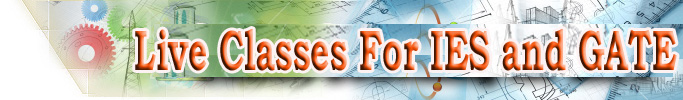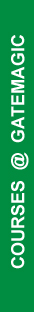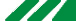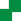﻿ IESGATE.COM - The Official Website
 Join  our Virtual Interactive Classroom NOW! Try out our Sample Test Watch our Sample Video Attend our  Orientation Programme Call: +91 9000018804Home | Institute | GATE | IES | Virtual Classroom | Courses | Faculty | Resources | Feedbacks | Contact UsCOURSES - GATE - Electronics and Communication Engineering
Course Summary

 Duration: 150 hours Faculty: XXXX Course Fee: Rs. 12000 -/- Course Features: Online classroom sessions (150 hours) Practice test (one topicwise test) Mock test (2) Join: Contact For any further queries regarding the course, contact Phone: +91 9000018804 Email: gate.ee@gatemagic.com

 Course Details Faculty Syllabus Online Classroom Sessions Tests Timetable Recommended Books / References Popularity as an optional subject Preparation Tips Joining the course Feedbacks

Faculty

The GATE - Electronics and Communication Engineering course is handled by XXXX.

Syllabus

ENGINEERING MATHEMATICS

• Linear Algebra: Matrix Algebra, Systems of linear equations, Eigen values and eigen vectors.
• Calculus: Mean value theorems, Theorems of integral calculus, Evaluation of definite and improper integrals, Partial Derivatives, Maxima and minima, Multiple integrals, Fourier series. Vector identities, Directional derivatives, Line, Surface and Volume integrals, Stokes, Gauss and Green's theorems.
• Differential equations: First order equation (linear and nonlinear), Higher order linear differential equations with constant coefficients, Method of variation of parameters, Cauchy's and Euler's equations, Initial and boundary value problems, Partial Differential Equations and variable separable method.
• Complex variables: Analytic functions, Cauchy's integral theorem and integral formula, Taylor's and Laurent' series, Residue theorem, solution integrals.
• Probability and Statistics: Sampling theorems, Conditional probability, Mean, median, mode and standard deviation, Random variables, Discrete and continuous distributions, Poisson,Normal and Binomial distribution, Correlation and regression analysis.
• Numerical Methods: Solutions of non-linear algebraic equations, single and multi-step methods for differential equations.
• Transform Theory: Fourier transform,Laplace transform, Z-transform.
ELECTRONICS AND COMMUNICATION ENGINEERING
• Networks: Network graphs: matrices associated with graphs; incidence, fundamental cut set and fundamental circuit matrices. Solution methods: nodal and mesh analysis. Network theorems: superposition, Thevenin and Norton's maximum power transfer, Wye-Delta transformation. Steady state sinusoidal analysis using phasors. Linear constant coefficient differential equations; time domain analysis of simple RLC circuits, Solution of network equations usingLaplace transform: frequency domain analysis of RLC circuits. 2-port network parameters: driving point and transfer functions. State equations for networks.
• Electronic Devices: Energy bands in silicon, intrinsic and extrinsic silicon. Carrier transport in silicon: diffusion current, drift current, mobility, and resistivity. Generation and recombination of carriers.p-n junction diode, Zener diode, tunnel diode, BJT, JFET, MOS capacitor, MOSFET, LED, p-I-n and avalanche photo diode, Basics of LASERs. Device technology: integrated circuits fabrication process, oxidation, diffusion, ion implantation, photolithography, n-tub, p-tub and twin-tub CMOS process.
• Analog Circuits: Small Signal Equivalent circuits of diodes, BJTs, MOSFETs and analog CMOS. Simple diode circuits, clipping, clamping, rectifier.Biasing and bias stability of transistor and FET amplifiers. Amplifiers: single-and multi-stage, differential and operational, feedback, and power. Frequency response of amplifiers.Simple op-amp circuits. Filters. Sinusoidal oscillators; criterion for oscillation; single-transistor and op-amp configurations.Function generators and wave-shaping circuits, 555 Timers. Power supplies.
• Digital circuits: Boolean algebra, minimization of Boolean functions; logic gates; digital IC families (DTL, TTL, ECL, MOS, CMOS). Combinatorial circuits: arithmetic circuits, code converters, multiplexers, decoders, PROMs and PLAs. Sequential circuits: latches and flip-flops, counters and shift-registers. Sample and hold circuits, ADCs, DACs. Semiconductor memories. Microprocessor(8085): architecture, programming, memory and I/O interfacing.
• Signals and Systems: Definitions and properties ofLaplace transform, continuous-time and discrete-time Fourier series, continuous-time and discrete-time Fourier Transform, DFT and FFT, z-transform. Sampling theorem. Linear Time-Invariant (LTI) Systems: definitions and properties; causality, stability, impulse response, convolution, poles and zeros, parallel and cascade structure, frequency response, group delay, phase delay. Signal transmission through LTI systems.
• Control Systems: Basic control system components; block diagrammatic description, reduction of block diagrams. Open loop and closed loop (feedback) systems and stability analysis of these systems. Signal flow graphs and their use in determining transfer functions of systems; transient and steady state analysis of LTI control systems and frequency response. Tools and techniques for LTI control system analysis: root loci, Routh-Hurwitz criterion, Bode and Nyquist plots. Control system compensators: elements of lead and lag compensation, elements of Proportional-Integral-Derivative (PID) control. State variable representation and solution of state equation of LTI control systems.
• Communications: Random signals and noise: probability, random variables, probability density function, autocorrelation, power spectral density. Analog communication systems: amplitude and angle modulation and demodulation systems, spectral analysis of these operations, superheterodyne receivers; elements of hardware, realizations of analog communication systems; signal-to-noise ratio (SNR) calculations for amplitude modulation (AM) and frequency modulation (FM) for low noise conditions. Fundamentals of information theory and channel capacity theorem. Digital communication systems: pulse code modulation (PCM), differential pulse code modulation (DPCM), digital modulation schemes: amplitude, phase and frequency shift keying schemes (ASK, PSK, FSK), matched filter receivers, bandwidth consideration and probability of error calculations for these schemes. Basics of TDMA, FDMA and CDMA and GSM.
• Electromagnetics: Elements of vector calculus: divergence and curl; Gauss' and Stokes' theorems, Maxwell's equations: differential and integral forms. Wave equation, Poynting vector. Plane waves: propagation through various media; reflection and refraction; phase and group velocity; skin depth. Transmission lines: characteristic impedance; impedance transformation; Smith chart; impedance matching; S parameters, pulse excitation. Waveguides: modes in rectangular waveguides; boundary conditions; cut-off frequencies; dispersion relations. Basics of propagation in dielectric waveguide and optical fibers. Basics of Antennas: Dipole antennas; radiation pattern; antenna gain.

Online Classroom Sessions

• The course comprises of online classroom sessions for a duration of 150 hours.
• Click here to check the weekly timetable schedule for this course.

Tests Timetable

• A total of one subjectwise / topicwise practice test each and 2 Mock tests is scheduled for this course.

Recommended Books / References

The following are the suggested books / references that the students can consult while preparing for GATE - Electronics and Communication Engineering.

• A
• A
• A

Popularity as an optional subject

The following are the reasons why this subject is popular among students.

• a
• a
• a

Preparation Tips

The following are simple preparation tips to score well in the GATE - Electronics and Communication Engineering.

• a
• a
• a

Joining the course

Regular Student

• Students can register as a regular user for any of the courses offered by IESGATE.COM by making the full payment for the course online.
• As a regular user, students can
• Participate in virtual classroom sessions and directly interact with the faculty and students during the online coaching classes.
• Gain access to the Comprehensive Question Bank until the final examination.
• Gain access to the pre-recorded videos of the registered courses until the final examination.
• Participate in the Practice / Mock tests conducted during the course.
• Registered students would receive intimation about the scheduled virtual classroom sessions on their registered mobile and email ids. Further, an automated reminder would be sent to the registered mobile number 10 minutes before the online session.

Trial User:
• Students can register for a trial period of one week for any of the courses offered by IESGATE.COM (except comprehensive coaching) by paying a nominal fee of Rs. 500.
• As a trial user, students can
• Participate in 3 to 5 virtual classroom session for any subject of their choice and directly interact with the faculty and students participating in the session.
• Gain access to the Comprehensive Question Bank for a period of one week, post which the trial period will expire.
• Trial users can participate in the Practice / Mock tests conducted during this period.
• Registered students would receive intimation about the scheduled virtual classroom sessions on their registered mobile and email ids. Further, an automated reminder would be sent to the registered mobile number 10 minutes before the online session.

Feedbacks

 What our students say... Display random from DB... for this subject - Chandan Kumar Singh, Reservoir Engineer Reliance Industries Ltd., Mumbai Read More...